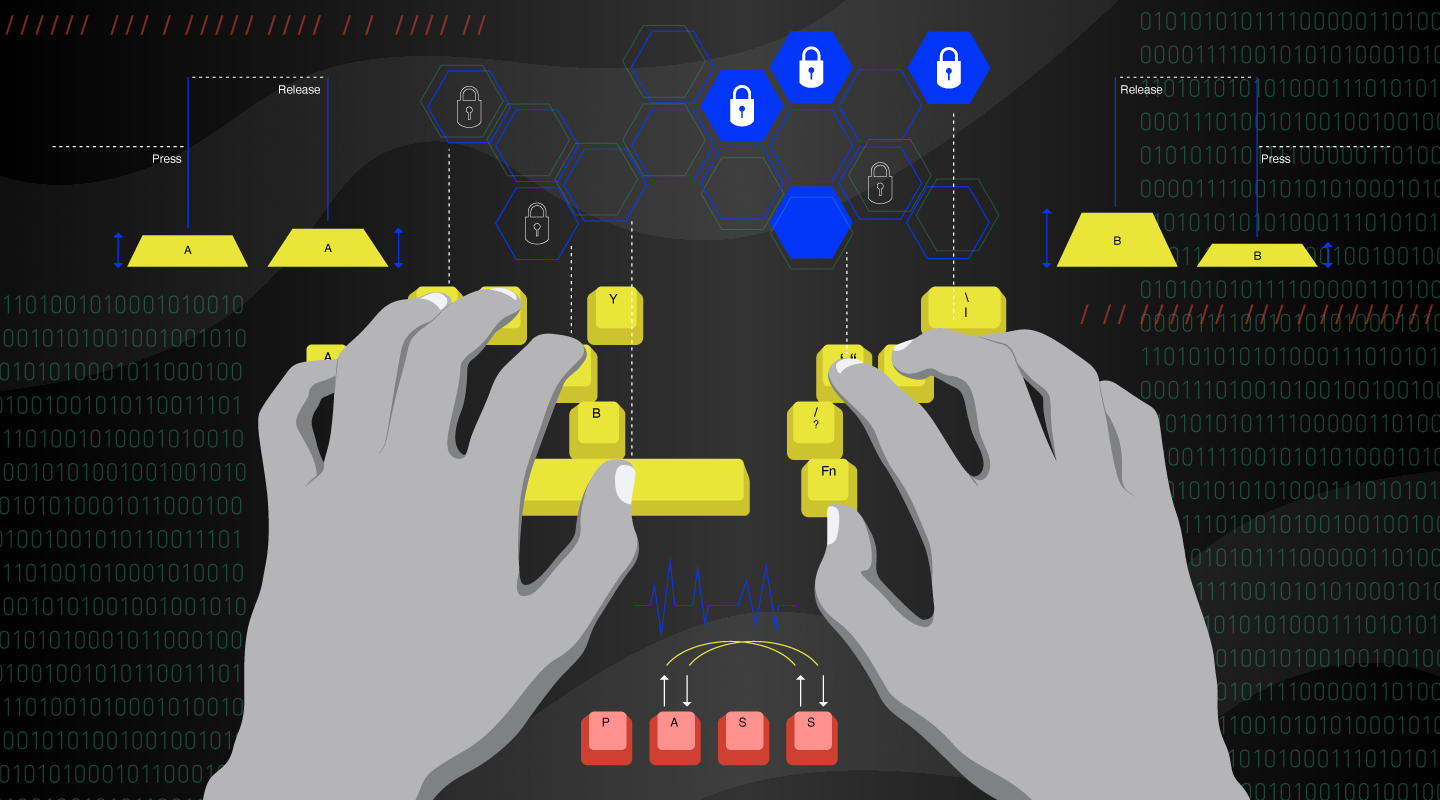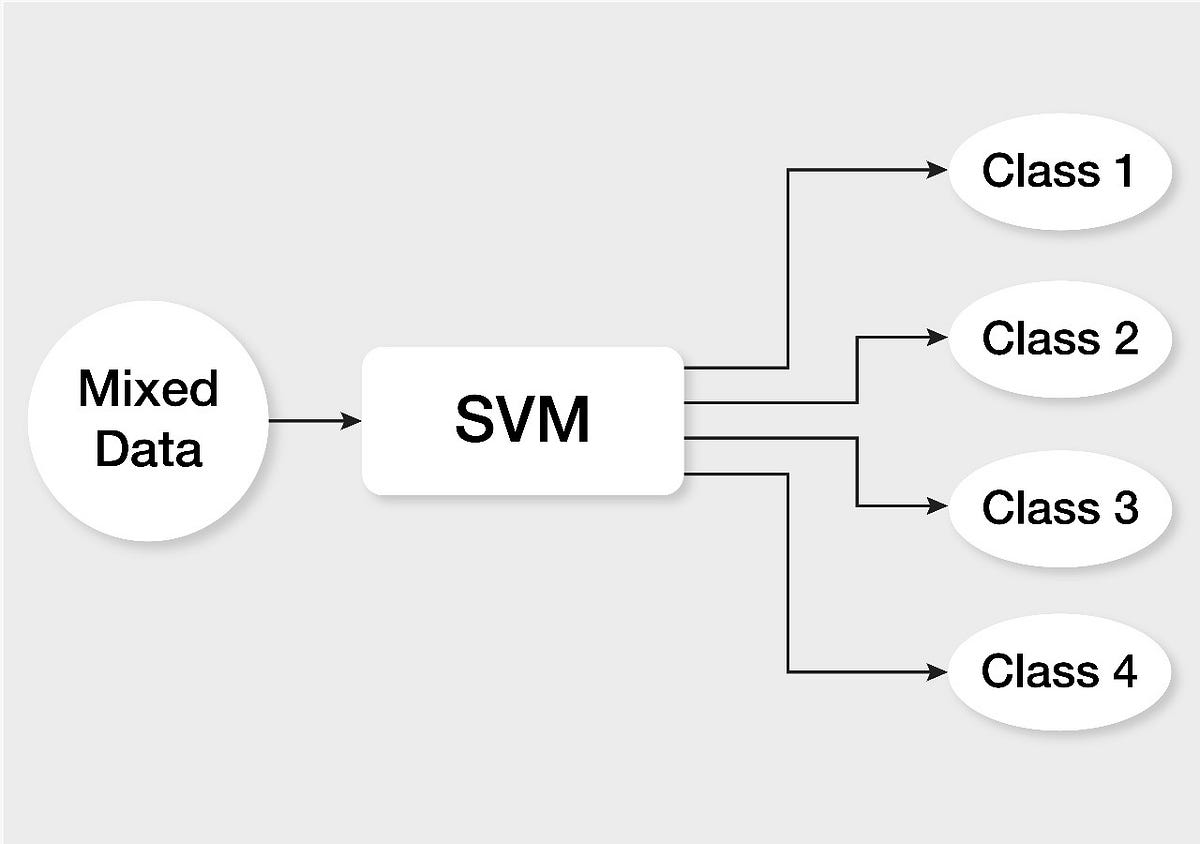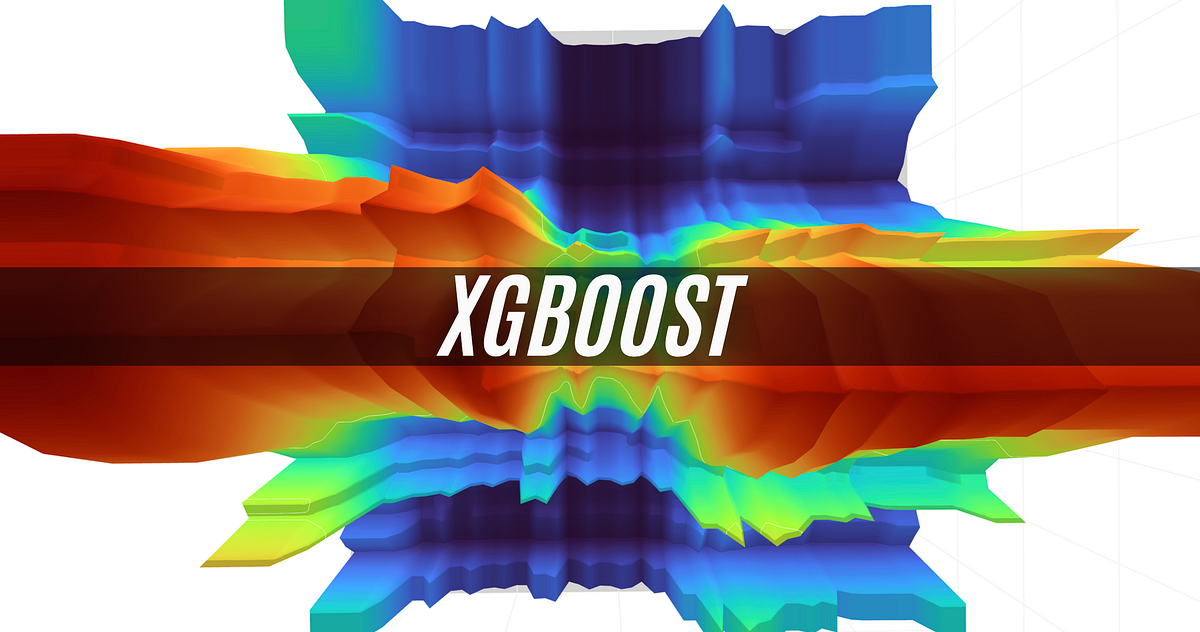# The Application of ML Models in User Recognition via Keystroke Dynamics

Published on
11-10-2023
Author
Aisys
Category
News## ML models for User Recognition using Keystroke Dynamics

The keystroke dynamics that are used in this article’s machine learning models for user recognition are behavioral biometrics. Keystroke dynamics use the distinctive way that each person types to confirm their identity. This is accomplished by analyzing the 2 keystroke events on Key-Press and Key-Release — that make up a keystroke on computer keyboards to extract typing patterns.

This article will examine how these patterns can be applied to create 3 precise machine-learning models for user recognition.The goal of this article will be split into two parts, building and training Machine Learning models (1. SVM 2. Random Forest 3. XGBoost) and deploying the model in a real live single point API capable of predicting the user based on 5 input parameters: the ML model and 4 keystroke times.

## 1. Building Models

### The problem

The objective of this part is to build ML models for user recognition based on their keystroke data. keystroke dynamics is a behavioral biometric that utilizes the unique way a person types to verify the identity of an individual.

Typing patterns are predominantly extracted from computer keyboards. the patterns used in keystroke dynamics are derived mainly from the two events that make up a keystroke: the Key-Press and Key-Release.

The Key-Press event takes place at the initial depression of a key and the Key-Release occurs at the subsequent release of that key.

In this step, a dataset of keystroke information of users is given with the following information:

• `keystroke.csv`: in this dataset, the keystroke data from 110 users are collected.
• All users are asked to type a 13-length constant string 8 times and the keystroke data (key-press time and key-release time for each key) are collected.
• The data set contains 880 rows and 27 columns.
• The first column indicates UserID and the rest shows the press and release time for the first to 13th character.

### You should do the following:

1. Usually, the raw data is not informative enough, and it is needed to extract informative features from raw data to build a good model.

In this regard, four features:

• `Hold Time “HT”`

• `Press-Press time “PPT”`

• `Release-Release Time “RRT”`

• `Release-Press time “RPT”`

are introduced and the definitions of each of them are described above.

2. For each row in `keystroke.csv,` you should generate these features for each two consecutive keys.

3. After completing the previous step, you should calculate the mean and standard deviation for each feature per row. As a result, you should have 8 features (4 mean and 4 standard deviations) per row. → `process_csv()`

``````def calculate_mean_and_standard_deviation(feature_list):
from math import sqrt
# calculate the mean
mean = sum(feature_list) / len(feature_list)

# calculate the squared differences from the mean
squared_diffs = [(x - mean) ** 2 for x in feature_list]

# calculate the sum of the squared differences
sum_squared_diffs = sum(squared_diffs)

# calculate the variance
variance = sum_squared_diffs / (len(feature_list) - 1)

# calculate the standard deviation
std_dev = sqrt(variance)

return mean, std_dev
``````

``````def process_csv(df_input_csv_data):
data = {
'user': [],
'ht_mean': [],
'ht_std_dev': [],
'ppt_mean': [],
'ppt_std_dev': [],
'rrt_mean': [],
'rrt_std_dev': [],
'rpt_mean': [],
'rpt_std_dev': [],
}

# iterate over each row in the dataframe
for i, row in df_input_csv_data.iterrows():
# iterate over each pair of consecutive presses and releases
# print('user:', row['user'])

# list of hold times
ht_list = []
# list of press-press times
ppt_list = []
# list of release-release times
rrt_list = []
# list of release-press times
rpt_list = []

# I use the IF to select only the X rows  of the csv
if i < 885:
for j in range(12):
# calculate the hold time: release[j]-press[j]
ht = row[f"release-{j}"] - row[f"press-{j}"]
# append hold time to list of hold times
ht_list.append(ht)

# calculate the press-press time: press[j+1]-press[j]
if j < 11:
ppt = row[f"press-{j + 1}"] - row[f"press-{j}"]
ppt_list.append(ppt)

# calculate the release-release time: release[j+1]-release[j]
if j < 11:
rrt = row[f"release-{j + 1}"] - row[f"release-{j}"]
rrt_list.append(rrt)

# calculate the release-press time: press[j+1] - release[j]
if j < 10:
rpt = row[f"press-{j + 1}"] - row[f"release-{j}"]
rpt_list.append(rpt)

# ht_list, ppt_list, rrt_list, rpt_list are a list of calculated values for each feature -> feature_list
ht_mean, ht_std_dev = calculate_mean_and_standard_deviation(ht_list)
ppt_mean, ppt_std_dev = calculate_mean_and_standard_deviation(ppt_list)
rrt_mean, rrt_std_dev = calculate_mean_and_standard_deviation(rrt_list)
rpt_mean, rpt_std_dev = calculate_mean_and_standard_deviation(rpt_list)
# print(ht_mean, ht_std_dev)
# print(ppt_mean, ppt_std_dev)
# print(rrt_mean, rrt_std_dev)
# print(rpt_mean, rpt_std_dev)

data['user'].append(row['user'])
data['ht_mean'].append(ht_mean)
data['ht_std_dev'].append(ht_std_dev)
data['ppt_mean'].append(ppt_mean)
data['ppt_std_dev'].append(ppt_std_dev)
data['rrt_mean'].append(rrt_mean)
data['rrt_std_dev'].append(rrt_std_dev)
data['rpt_mean'].append(rpt_mean)
data['rpt_std_dev'].append(rpt_std_dev)

else:
break
data_df = pd.DataFrame(data)
return data_df
``````

All the code you can find on my GitHub in the KeystrokeDynamics repository:GITHUB

### GitHub - BogdanAlinTudorache/KeystrokeDynamics: This repository focuses on the use of keystroke dynamics as a behavioral biometric to build machine learning models for user recognition.

This repository focuses on the use of keystroke dynamics as a behavioral biometric to build machine learning models for user recognition. - GitHub - BogdanAlinTudorache/KeystrokeDynamics: This rep...

## Train the MLs

Now that we have parsed the data we can start building the models by training the MLs.

### Support Vector Machine

``````
def train_svm(training_data, features):
import joblib
from sklearn.svm import SVC

"""
SVM stands for Support Vector Machine, which is a type of machine learning algorithm used:
for classification and regression analysis.

SVM algorithm aims to find a hyperplane in an n-dimensional space that separates the data into two classes.
The hyperplane is chosen in such a way that it maximizes the margin between the two classes,
making the classification more robust and accurate.

In addition, SVM can also handle non-linearly separable data by mapping the original features to a
higher-dimensional space, where a linear hyperplane can be used for classification.

:param training_data:
:param features:
:return: ML Trained model
"""

# Split the data into features and labels
X = training_data[features]
y = training_data['user']

# Train an SVM model on the data
svm_model = SVC()
svm_model.fit(X, y)

# Save the trained model to disk
svm_model_name = 'models/svm_model.joblib'
joblib.dump(svm_model, svm_model_name)
``````MEDIUM

### Support Vector Machines(SVM) — An Overview

Machine learning involves predicting and classifying data and to do so we employ various machine learning algorithms according to the…

## Random Forest

``````def train_random_forest(training_data, features):
"""
Random Forest is a type of machine learning algorithm that belongs to the family of ensemble learning methods.
It is used for classification, regression, and other tasks that involve predicting an output value based on
a set of input features.

The algorithm works by creating multiple decision trees, where each tree is built using a random subset of the
input features and a random subset of the training data. Each tree is trained independently,
and the final output is obtained by combining the outputs of all the trees in some way, such as taking the average
(for regression) or majority vote (for classification).

:param training_data:
:param features:
:return: ML Trained model
"""
import joblib
from sklearn.ensemble import RandomForestClassifier

# Split the data into features and labels
X = training_data[features]
y = training_data['user']

# Train a Random Forest model on the data
rf_model = RandomForestClassifier()
rf_model.fit(X, y)

# Save the trained model to disk
rf_model_name = 'models/rf_model.joblib'
joblib.dump(rf_model, rf_model_name)
``````MEDIUM

### Random Forest in Python

A Practical End-to-End Machine Learning Example

``````def train_xgboost(training_data, features):
import joblib
import xgboost as xgb
from sklearn.preprocessing import LabelEncoder
"""
XGBoost stands for Extreme Gradient Boosting, which is a type of gradient boosting algorithm used for classification and regression analysis.
XGBoost is an ensemble learning method that combines multiple decision trees to create a more powerful model.
Each tree is built using a gradient boosting algorithm, which iteratively improves the model by minimizing a loss function.
XGBoost has several advantages over other boosting algorithms, including its speed, scalability, and ability to handle missing values.

:param training_data:
:param features:
:return: ML Trained model
"""

# Split the data into features and labels
X = training_data[features]
label_encoder = LabelEncoder()
y = label_encoder.fit_transform(training_data['user'])

# Train an XGBoost model on the data
xgb_model = xgb.XGBClassifier()
xgb_model.fit(X, y)

# Save the trained model to disk
xgb_model_name = 'models/xgb_model.joblib'
joblib.dump(xgb_model, xgb_model_name)
``````MEDIUM

### XGBoost: Extreme Gradient Boosting — How to Improve on Regular Gradient Boosting?

A detailed look at differences between the two algorithms and when you should choose one over the other

Also published here.

If you like the article and would like to support me, make sure to: